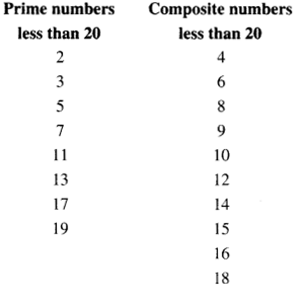# NCERT Solutions for Class 6 Maths Chapter 3 Playing With Numbers Ex 3.2

NCERT Solutions for Class 6 Maths Chapter 3 Playing With Numbers Ex 3.2 are part of NCERT Solutions for Class 6 Maths. Here we have given NCERT Solutions for Class 6 Maths Chapter 3 Playing With Numbers Ex 3.2.

 Board CBSE Textbook NCERT Class Class 6 Subject Maths Chapter Chapter 3 Chapter Name Playing With Numbers Exercise Ex 3.2 Number of Questions Solved 12 Category NCERT Solutions

## NCERT Solutions for Class 6 Maths Chapter 3 Playing With Numbers Ex 3.2

Question 1.
What is the sum of any two :
(a) Odd numbers
(b) Even numbers.
Solution :
(a) The sum of any two odd numbers is an even number.
(b) The sum of any two even numbers is an even number.

Question 2.
State whether the following statements are True or False :

1. The sum of three odd numbers is even.
2. The sum of two odd numbers and one even number is even.
3. The product of three odd numbers is odd.
4. If an even number is divided by 2, the quotient is always odd.
5. All prime numbers are odd.
6. Prime numbers do not have any factors.
7. Sum of two prime numbers is always even.
8. 2 is the only even prime number.
9. All even numbers are composite numbers.
10. The product of two even numbers is always even.

Solution :

1. This statement is false.
2. This statement is true.
3. This statement is true.
4. This statement is false.
5. This statement is false.
6. This statement is false.
7. This statement is false.
8. This statement is true.
9. This statement is false.
10. This statement is true.

Question 3.
The numbers 13 and 31 are prime numbers. Both these numbers have the same digits 1 and 3. Find such pairs of prime numbers up to 100.
Solution :
All other such pairs of prime numbers up to 100 are as follows :
17 and 71; 37 and 73; 79 and 97.

Question 4.
Write down separately the prime and composite numbers less than 20.
Solution :Question 5.
What is the greatest prime number between 1 and 10?
Solution :
The greatest prime number between 1 and 10 is 7.

Question 6.
Express the following as the sum of two odd primes :
(a) 44
(b) 36
(c) 24
(d) 18.
Solution :
(a) 44 = 3+41
(b) 36 = 5 + 31
(c) 24 = 5+19
(d) 18 = 5+13.

Question 7.
Give three pairs of prime numbers whose difference is 2.
[Remark: Two prime numbers whose difference is 2 are called twin primes],
Solution :
The three pairs of prime numbers, whose difference is 2, are as follows :
3, 5 ; 5, 7 ; 11, 13.

Question 8.
Which of the following numbers are prime?
(a) 23
(b) 51
(c) 37
(d) 26.
Solution :
(a) 23,
(c) 37 are prime numbers.

Question 9.
Write seven consecutive composite numbers less than 100 so that there is no prime number between them.
Solution :
90, 91, 92, 93, 94, 95, 96.

Question 10.
Express each of the following numbers as the sum of three odd primes :
(a) 21
(b) 31
(c) 53
(d) 61.
Solution :
(a) 21 = 3 + 5 + 13
(b) 31 = 3 + 5 + 23
(c) 53 = 13+ 17 + 23
(d) 61 =7;+ 13+41.

Question 11.
Write five pairs of prime numbers below 20 whose sum is divisible by 5. (Hint: 3 + 7 – 10).
Solution :
2, 3 ; 2, 13 ; 3, 17 ; 7, 13 ; 11, 19.

Question 12.
Fill in the blanks in the following :

1. A number which has only two factors is called a
2. A number which has more than two factors is called a
3. 1 is neither nor
4. The smallest prime number is
5. The smallest composite number is
6. The smallest even number is

Solution :

1. A number which has only two factors is called a prime number.
2. A number which has more than two factors is called a composite number.
3. 1 is neither prime number nor composite number.
4. The smallest prime number is 2.
5. The smallest composite number is 4.
6. The smallest even number is 2.

We hope the NCERT Solutions for Class 6 Maths Chapter 3 Playing With Numbers Ex 3.2 help you. If you have any query regarding NCERT Solutions for Class 6 Maths Chapter 3 Playing With Numbers Ex 3.2, drop a comment below and we will get back to you at the earliest.